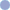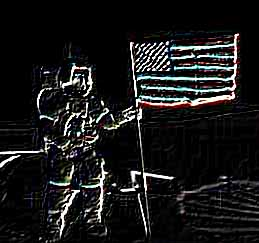ConvolutionThe Convolution Effect allows you to produce a range of effects by specifying a set of convolution kernels. A simple explanation is given here but you may wish to refer to other sources for complete descriptions of convolution and how you can use it.
 SettingsName Default DescriptionWidth 3 pixels The width of the median filter to be applied.Height 3 pixels The height of the median filter to be applied.X 2 pixels The horizontal center of the filter in pixels from top left.Y 2 pixels The vertical center of the filter in pixels from top left.Number 2 The number of kernels to apply.Values See desc. The values for the kernels in a comma delimited list. The items in the list may be integers or floating point numbers. The default value is: "-1,0,1,-2,0,2,-1,0,1,1,2,1,0,0,0,-1,-2,-1"How it WorksConvolution is a general purpose filter effect for images. It works by determining the value of a central pixel by adding the weighted values of all its neighbors together. The weights applied to each pixel are determined by what is called a convolution kernel.

So if you want to take the average of all the immediate neighbors of a central pixel you would specify an equally weighted convolution kernel. Note that the total sum of all the weights is one so that the overall brightness of the image is not affected by the convolution. Note that the anchor point is highlighted to show which pixel should be regarded as central.0.1111 0.1111 0.11110.1111 0.1112 0.11110.1111 0.1111 0.1111If you wanted to take the average of the pixels immediately above, below and to the sides of the central pixel, and you wanted to exclude the central pixel itself, you would specify the following kernel.0.0000 0.2500 0.00000.2500 0.0000 0.25000.0000 0.2500 0.0000Although a three square kernel with the anchor at the center is most common, you can use other shapes of kernel. For example the following convolution will shift the entire image left by one pixel.0.0000 1.0000When you specify values you specify them from left to right and then from top to bottom. You can specify more than one filter at a time and the results will be added together.

The default kernel is a standard Sobel edge detector. This contains two filters - one vertical, one horizontal - to be applied and then added together. The two kernel values are:-1 0 1-2 0 2-1 0 11 2 10 0 0-1 -2 -1ExampleThe following examples show the effect of the default kernel - a Sobel Edge Detector.Original ImageAfter Convolution Applied